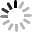Events

# Calculate the load capacity coefficient of caster wheels

March 24, 2021Calculate the load-bearing weight: In order to be able to calculate the required load capacity of various casters, it is necessary to know the weight of the transport equipment, the maximum load and the number of wheels and casters used. The required load capacity of a single wheel or caster is calculated as follows：

T=(E+Z)/M×N:

---T=The required weight of a single wheel or caster;

---E=The weight of the transportation equipment;